## Homework 9

1. An electron in the Hydrogen potentialis in the state. Find the value ofthat properly normalizes the state. What is the probability that the electron be found in the ground state of Hydrogen?

2. An electron is in thestate of hydrogen. Find its wave function in momentum space.

3. A spinparticle is in an eigenstate of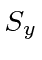with eigenvalue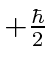at time. At that time it is placed in a constant magnetic fieldin the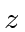direction. The spin is allowed to precess for a time. At that instant, the magnetic field is very quickly switched to thedirection. After another time interval, a measurement of thecomponent of the spin is made. What is the probability that the value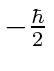will be found?

4. Consider a system of spin. What are the eigenstates and eigenvalues of the operator? Suppose a measurement of this quantity is made, and the system is found to be in the eigenstate with the larger eigenvalue. What is the probability that a subsequent measurement ofyields?

5. Let's define the u axis to be in the x-z plane, between the positive x and z axes and at an angle of 30 degrees to the x axis. Given an unpolarized spinbeam of intensity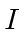going into the following Stern-Gerlach apparati, what intensity comes out?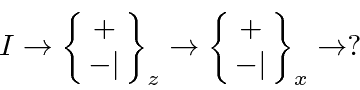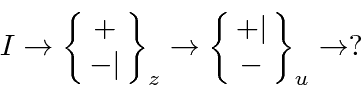Jim Branson 2013-04-22